﻿ Python Tkinter dawing Program with shapes and text

# Creating a versatile drawing program with Python and tkinter

## Python Tkinter Canvas and Graphics: Exercise-7 with Solution

Write a Python program that creates a drawing program with Tkinter that supports freehand drawing, shapes, and text annotations on the Canvas.

Sample Solution:

Python Code:

``````import tkinter as tk
from tkinter import ttk

class DrawingApp:
def __init__(self, root):
self.root = root
self.root.title("Drawing App")

# Create a canvas
self.canvas = tk.Canvas(root, bg="white")
self.canvas.pack(fill=tk.BOTH, expand=True)

# Create a toolbar
self.toolbar = ttk.Frame(root)
self.toolbar.pack(side=tk.TOP, fill=tk.X)

# Create drawing tools buttons
self.pen_button = ttk.Button(self.toolbar, text="Pen", command=self.use_pen)
self.pen_button.grid(row=0, column=0)

self.line_button = ttk.Button(self.toolbar, text="Line", command=self.use_line)
self.line_button.grid(row=0, column=1)

self.rectangle_button = ttk.Button(self.toolbar, text="Rectangle", command=self.use_rectangle)
self.rectangle_button.grid(row=0, column=2)

self.text_button = ttk.Button(self.toolbar, text="Text", command=self.use_text)
self.text_button.grid(row=0, column=3)

# Initialize drawing mode
self.drawing_mode = "pen"

# Binding mouse events
self.canvas.bind("<Button-1>", self.start_drawing)
self.canvas.bind("<B1-Motion>", self.draw)
self.canvas.bind("<ButtonRelease-1>", self.stop_drawing)

# Variables for drawing
self.start_x, self.start_y = None, None
self.current_object = None

def use_pen(self):
self.drawing_mode = "pen"

def use_line(self):
self.drawing_mode = "line"

def use_rectangle(self):
self.drawing_mode = "rectangle"

def use_text(self):
self.drawing_mode = "text"

def start_drawing(self, event):
self.start_x, self.start_y = event.x, event.y
if self.drawing_mode == "pen":
self.current_object = self.canvas.create_line(event.x, event.y, event.x, event.y, fill="black", width=2)
elif self.drawing_mode == "text":
text = input("Enter text: ")
self.current_object = self.canvas.create_text(event.x, event.y, text=text, font=("Arial", 12))
else:
self.start_x, self.start_y = event.x, event.y

def draw(self, event):
if self.drawing_mode == "pen":
x, y = event.x, event.y
self.canvas.create_line(self.start_x, self.start_y, x, y, fill="black", width=2)
self.start_x, self.start_y = x, y
elif self.drawing_mode == "line":
if self.current_object:
self.canvas.delete(self.current_object)
self.current_object = self.canvas.create_line(self.start_x, self.start_y, event.x, event.y, fill="black", width=2)
elif self.drawing_mode == "rectangle":
if self.current_object:
self.canvas.delete(self.current_object)
self.current_object = self.canvas.create_rectangle(self.start_x, self.start_y, event.x, event.y, outline="black", width=2)
else:
pass

def stop_drawing(self, event):
self.current_object = None

if __name__ == "__main__":
root = tk.Tk()
app = DrawingApp(root)
root.mainloop()
```
```

The above exercise creates a basic drawing application with four drawing tools: pen, line, rectangle, and text. We can switch between drawing tools using the toolbar buttons and use the left mouse button to draw on the canvas. In this program, the user is shown how to handle different drawing modes and create various objects on the canvas.

Output:

```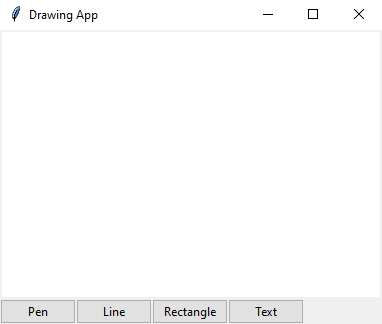```
```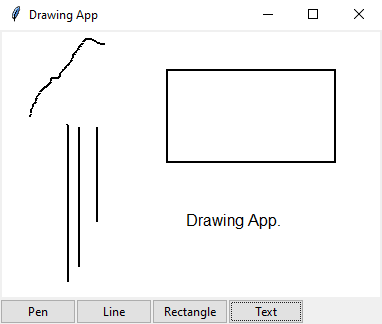```

Flowchart: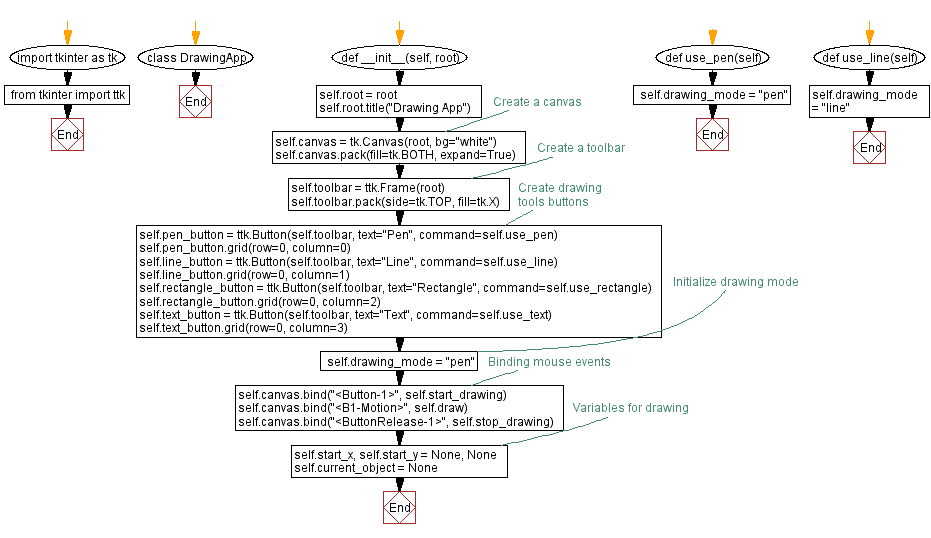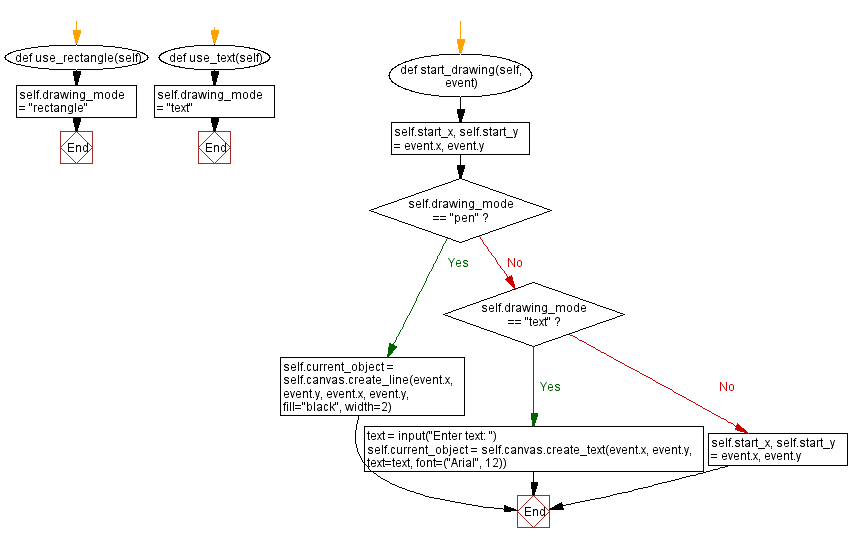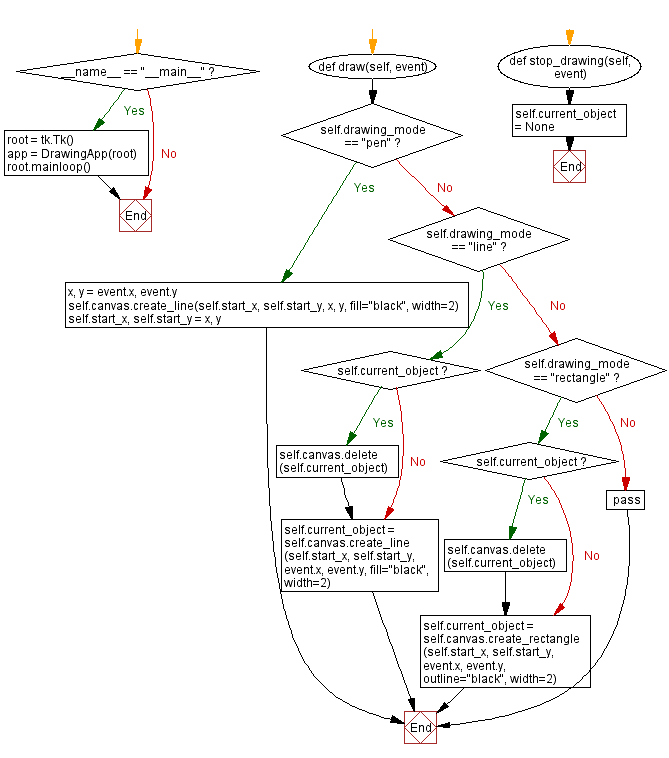Python Code Editor:

What is the difficulty level of this exercise?

Test your Programming skills with w3resource's quiz.

﻿

## Python: Tips of the Day

Summing a sequence of numbers (calculating the sum of zero to ten with skips):

```>>> l = range(0,10,2)
>>> sum(l)
20
```

We are closing our Disqus commenting system for some maintenanace issues. You may write to us at reach[at]yahoo[dot]com or visit us at Facebook